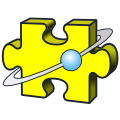BlackBox Component Builder

## Algebra

Computational Algebra library.
Depends on: Multi
Details:

Algebra is a 'Computational' algebra system, and can numerically evaluate elements from many mathematical structures. It is not a 'Computer Algebra System' that can handle these elements symbolically.

The modules fall into four classes:

AbstractExamples include Groups, Rings, & Fields as general ideas.
Semi-abstractVectors, Matrices, Rationals, Polynomials, & Rational functions.
The elements of these can be anything from the Abstract class.
(Since a polynomial is a ring, one can have polynomials of polynomials, etc.)
ConcreteSpecific examples of the abstract ideas.
Examples are 3-D Vectors, Gaussian Integers, Multi-precision complex numbers,Quaternions, and many others.
SpecialisedExamples are polynomial factorisation, orthogonal polynomials, type transfers.
PolyRoot can, for example, factorise complex polynomials to arbitrary precision.

AlgebraDemo gives an extensive demonstration of using the Algebra facilities.

Author: Robert D. Campbell